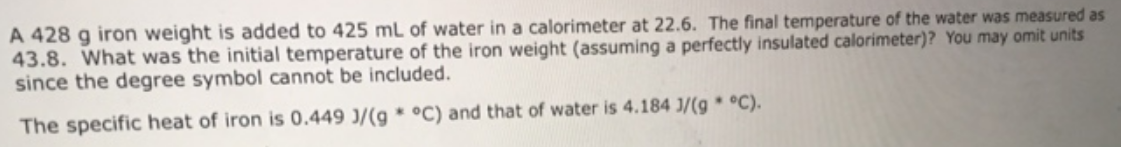# Problem: A 428 g iron weight is added to 425 ml of water in a calorimeter at 22.6. The final temperature of the water was measured as 43.8. What was the initial temperature of the iron weight (assuming a perfectly insulated calorimeter)? You may omit units since the degree symbol cannot be included. The specific heat of iron is 0.449 J/(g * °C) and that of water is 4,184 J/(g ° C).

###### FREE Expert Solution
95% (40 ratings)###### Problem Details

A 428 g iron weight is added to 425 ml of water in a calorimeter at 22.6. The final temperature of the water was measured as 43.8. What was the initial temperature of the iron weight (assuming a perfectly insulated calorimeter)? You may omit units since the degree symbol cannot be included. The specific heat of iron is 0.449 J/(g * °C) and that of water is 4,184 J/(g ° C).